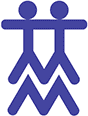# GeometryOur geometry program begins on the sensorial level with an introduction to the basic two-dimensional plane geometric shapes and the three-dimensional solids.  Through concrete materials such as the Geometry Cabinet, the Geometric Solids, the Geometry Sticks and the Constructive Triangles, students explore these shapes and their relationships to one another.  They also explore the concepts of point, line, plane and solid.  They are introduced to the plane and to parallel, divergent and convergent lines.  They also learn about the types of angles and their parts, the relationship between angles, intersections, plane figures and the construction of polygons.  Triangles and their terms are studied in depth.  The program continues with extensive work in the measurement, addition and subtraction of angles using both the Montessori and traditional protractors.  Students also do an in-depth study of quadrilaterals and regular and irregular polygons.  The circle and its parts are also introduced.Elementary GeometryThe 6 to 9 program culminates with a study of congruency, similarity and equivalency, using the Insets of Equivalency, and more advanced work with the Constructive Triangles, as well as an introduction to basic area work.  Students in the 9 to 12 program continue area work and are introduced to the Pythagorean Theorem, learning to apply the related formulae to abstract work.  They also complete a more in-depth study of circles, including work in figuring circumference and area, and they learn how to compute the surface area and volume of solids.  Our goal is to provide every child with a strong foundation in geometry through a concrete understanding of its concepts and applications in reality.